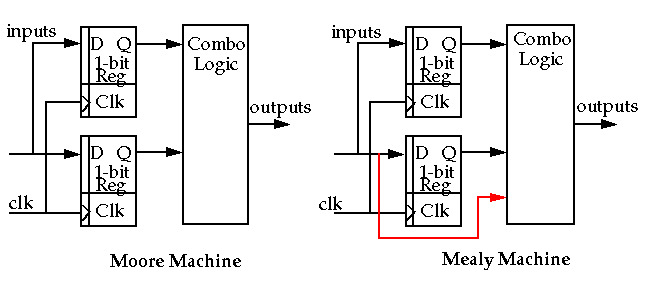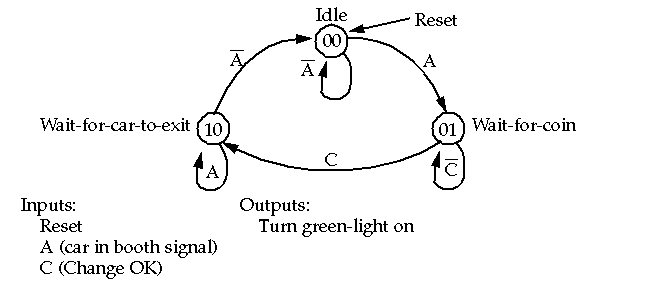## Control

• Control is the hard part of design.
• The regularity found in arithmetic and memory structures usually not present in control structures.

• Finite-State machines provide an organized structure for capturing control sequencing and operation.
• Two types of state machines:## Control

• State transition diagrams used to represent state machines.
• Toll-booth controller example:• Write the state equations of the form:
• if (state == oldstate & condition) next-state = newstate.
• Rules for assigning state bits and output encoding method given in Weste and Eshraghian.

## Control

• Control logic in CMOS is constructed in two main ways:
• Two-level sum-of-products
• Multilevel logic

• Two-level sum-of-products:
• PLA implementation:
• Regular structure for implementing combinational and sequential logic functions.
• We have examined how to construct combinational functions already.
• Sequential functions (finite-state machines) implementations are identical except that outputs are fed back to the inputs via registers.

• Pseudo n-MOS implementation main disadvantage is static power dissipation.

• Dynamic CMOS implementation involves suppling the AND plane and OR plane with clocks (see Weste and Eshraghian).

## Control

• Multilevel Logic:
• Most commonly used method for implementing control logic in CMOS.
• Cascaded groups of regular gates such as INVERTERS, BUFFERS, NANDs, NORs, XORs and AOIs.
•
• CAD systems are highly effective at automatically minimizing the logic, making state assignments and synthesizing layouts as gate-arrays or standard-cell layouts.
•